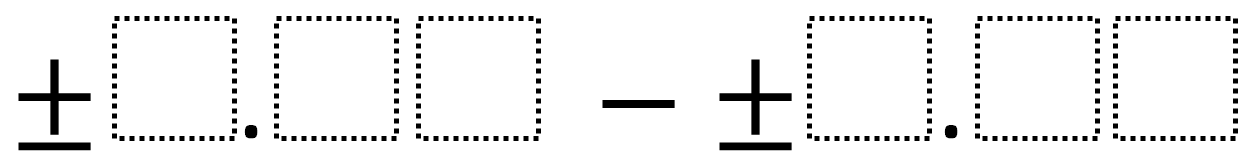# Subtracting Decimals (Middle School)

Directions: Using the digits 1 to 9 at most one time each, fill in the boxes to make the smallest (or largest) difference.### Hint

What number does each box represent?

No answers yet.  If you have one, post it in the comments.

Note: This problem’s difficulty can be adjusted by altering the number of digits (boxes), picking smallest or largest, or by picking either a positive, negative, or both.

Source: Robert Kaplinsky

## Greatest Common Factor

Directions: Using the digits 0 to 9 at most one time each, place a digit …

1.Subtracting decimals with integers (positive & negative) is not a 6th grade standard. That’s 7th grade.

•Robert Kaplinsky

Thanks for catching this Kristen. I’ll add 7.NS.1 to the problem.

2.Just considering positive number answers, using only a positive subtrahend and minuend for 6th grade, the greatest difference would be 8.64, obtained by taking the greatest possible positive number 9.87 and decreasing it by the smallest possible positive number, 1.23. Using only positive numbers again, the smallest possible difference would be 0.14 obtained by creating a mixed number with the greatest possible decimal value .98 and and subtracting it from a number whose ones place number is only 1 greater and whose decimal value is the smallest possible value .12. The possible subtrahend / minuend expressions woukd be: 7.12-6.98, 6.12-5.98, 5.12-4.98, and 4.12-3.98.

3.When having my 7th graders decide what integer sign to use the least value they could find was -9.65 – 8.74 = -18.39 or -9.75 – 8.64 = -18.39.